Olympiad Test Level 2: Quantitative Aptitude & Reasoning- 2

# Olympiad Test Level 2: Quantitative Aptitude & Reasoning- 2

Test Description

## 10 Questions MCQ Test GK Olympiad for Class 7 | Olympiad Test Level 2: Quantitative Aptitude & Reasoning- 2

Olympiad Test Level 2: Quantitative Aptitude & Reasoning- 2 for Class 7 2023 is part of GK Olympiad for Class 7 preparation. The Olympiad Test Level 2: Quantitative Aptitude & Reasoning- 2 questions and answers have been prepared according to the Class 7 exam syllabus.The Olympiad Test Level 2: Quantitative Aptitude & Reasoning- 2 MCQs are made for Class 7 2023 Exam. Find important definitions, questions, notes, meanings, examples, exercises, MCQs and online tests for Olympiad Test Level 2: Quantitative Aptitude & Reasoning- 2 below.
 1 Crore+ students have signed up on EduRev. Have you?
Olympiad Test Level 2: Quantitative Aptitude & Reasoning- 2 - Question 1

### Direction: There are four groups of letters in the following question. Of these, three are alike in some way and one of them is different. Identify the one that is different:

Detailed Solution for Olympiad Test Level 2: Quantitative Aptitude & Reasoning- 2 - Question 1

R, E,A,T appears is some order in all other words.

Olympiad Test Level 2: Quantitative Aptitude & Reasoning- 2 - Question 2

### Direction: In the question, first two given letter/number are related in some way. The same relation is in between third and fourth. Find out the correct answer among the alternatives. ACE:FGH::LNP?

Detailed Solution for Olympiad Test Level 2: Quantitative Aptitude & Reasoning- 2 - Question 2

The three letters of second word moved 5,4,3 and steps forward respectively.

Olympiad Test Level 2: Quantitative Aptitude & Reasoning- 2 - Question 3

### If in a certain code, HAT is 782, RABBIT is 681192. Then how will HABIT be coded as ?

Detailed Solution for Olympiad Test Level 2: Quantitative Aptitude & Reasoning- 2 - Question 3

Type - Direct substitution (Direct fashion)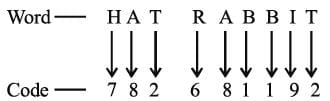Thus, the code for the given word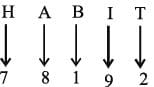Thus, option (b) is the correct answer.

Olympiad Test Level 2: Quantitative Aptitude & Reasoning- 2 - Question 4

In a class of 60, where girls are twice that of boys, Kamal ranked seventeenth from the top. If there are 9 girls ahead of Kamal, how many boys are after him in rank ?

Detailed Solution for Olympiad Test Level 2: Quantitative Aptitude & Reasoning- 2 - Question 4

Let the number of boys be x.
Then, number of girls = 2x.
∴ x + 2x = 60 or 3x = 60 or x=20.
So, number of boys = 20 and number of girls=40.
Number of students behind Kamal in rank (60-17) = 43.
Number of girls ahead of Kamal in rank= 9. Number of girls behind Kamal in rank = (40-9) = 31
∴ Number of boys behind Kamal in rank = (43-31)= 12.

Olympiad Test Level 2: Quantitative Aptitude & Reasoning- 2 - Question 5

Pointing towards a man in the photograph, lady said the father of his brother is the only son of my mother. How is the man related to lady ?

Detailed Solution for Olympiad Test Level 2: Quantitative Aptitude & Reasoning- 2 - Question 5

The father of his brother means “ his father” is the only son of my mother means “ my brother” It means lady’s is the father’s sister of the man’s father.

Olympiad Test Level 2: Quantitative Aptitude & Reasoning- 2 - Question 6

Jatin leaves his house and walks 12 km towards North. He turns right and walks another 12 km. He turns right again, walks 12 km more and turns left to walk 5 km. How far is he from his home and in which direction ?

Detailed Solution for Olympiad Test Level 2: Quantitative Aptitude & Reasoning- 2 - Question 6

(12 km + 5km = 17km)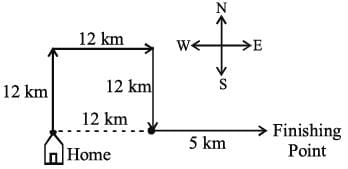Olympiad Test Level 2: Quantitative Aptitude & Reasoning- 2 - Question 7

What number should replace the question mark ?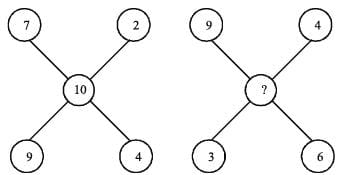Detailed Solution for Olympiad Test Level 2: Quantitative Aptitude & Reasoning- 2 - Question 7

Subtract the sum of the even numbers from the sum of the odd numbers.
7+9 = 16    9+3 = 12
2+4 = 6    4+6 = 10
16-6=10    12-10 = 2

Olympiad Test Level 2: Quantitative Aptitude & Reasoning- 2 - Question 8

Direction: Figures A and B are related in a particular manner. Establish the same relationship between Figures C and D by choosing a figure from amongst the alternatives, which would replace the question mark.

Problem Figures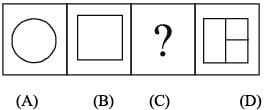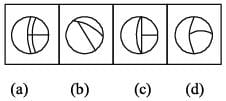Detailed Solution for Olympiad Test Level 2: Quantitative Aptitude & Reasoning- 2 - Question 8

From fig. (B) to fig. (A), the straight lines are converted to curved lines (or arcs).

Olympiad Test Level 2: Quantitative Aptitude & Reasoning- 2 - Question 9

Select from the alternative, the box that can be formed by folding the sheet shown in figure (X).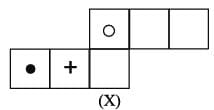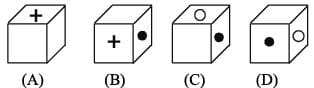Detailed Solution for Olympiad Test Level 2: Quantitative Aptitude & Reasoning- 2 - Question 9

When the sheet in fig.(X) is folded to form a cube, then the face bearing a dot appears opposite to a blank face, the face bearing a “+’ sign appears opposite to another blank face and the face bearing a circle appears opposite to the third blank face. Clearly, all the four cubes shown in figures (A), (B), (C) and (D) can be formed.

Olympiad Test Level 2: Quantitative Aptitude & Reasoning- 2 - Question 10

Direction: In the following examples there is a diagram marked (X), with one or more dots placed in it. The diagram is followed by four other figures, marked (a), (b), (c) and (d) only one of which is such as to make possible the placement of the alternative in each of these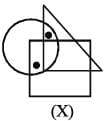Detailed Solution for Olympiad Test Level 2: Quantitative Aptitude & Reasoning- 2 - Question 10

In figure (X), one of file dots lies in the region common to the circle and the triangle only and the other dot lies in the region common to die circle and die square only. In figures (a), (c) and (d), the region common to the circle and the triangle lies within the square. Only figure (b) contains a region common to the circle and the triangle only and also a region common to the circle and the square.

## GK Olympiad for Class 7

63 tests
 Use Code STAYHOME200 and get INR 200 additional OFF Use Coupon Code
Information about Olympiad Test Level 2: Quantitative Aptitude & Reasoning- 2 Page
In this test you can find the Exam questions for Olympiad Test Level 2: Quantitative Aptitude & Reasoning- 2 solved & explained in the simplest way possible. Besides giving Questions and answers for Olympiad Test Level 2: Quantitative Aptitude & Reasoning- 2, EduRev gives you an ample number of Online tests for practice

63 tests